DAX offers two functions to retrieve the list of values of a column: VALUES and DISTINCT. The difference between the two is subtle. To understand it better, we first need to introduce the concept of the blank row. The blank row is a special row added to a table, in case the table is on the one-side of a strong relationship and the relationship is invalid. Let us elaborate on this.

Consider two measures to compute the number of colors, using the two functions VALUES and DISTINCT:

```#Colors Distinct := COUNTROWS ( DISTINCT ( 'Product'[Color] ) )
#Colors Values := COUNTROWS ( VALUES ( 'Product'[Color] ) )
```

The projection of these two measures in a matrix shows identical values, both on individual rows of the matrix and at the grand total.The two functions seem identical. The reason is that right now, all the relationships in the data model are in good shape. To notice a difference between DISTINCT and VALUES, we need to invalidate the relationship between Sales and Product. We can do this by removing a few products from the Product table. For example, we removed all the product with the pink color. This is the result after deleting the pink products: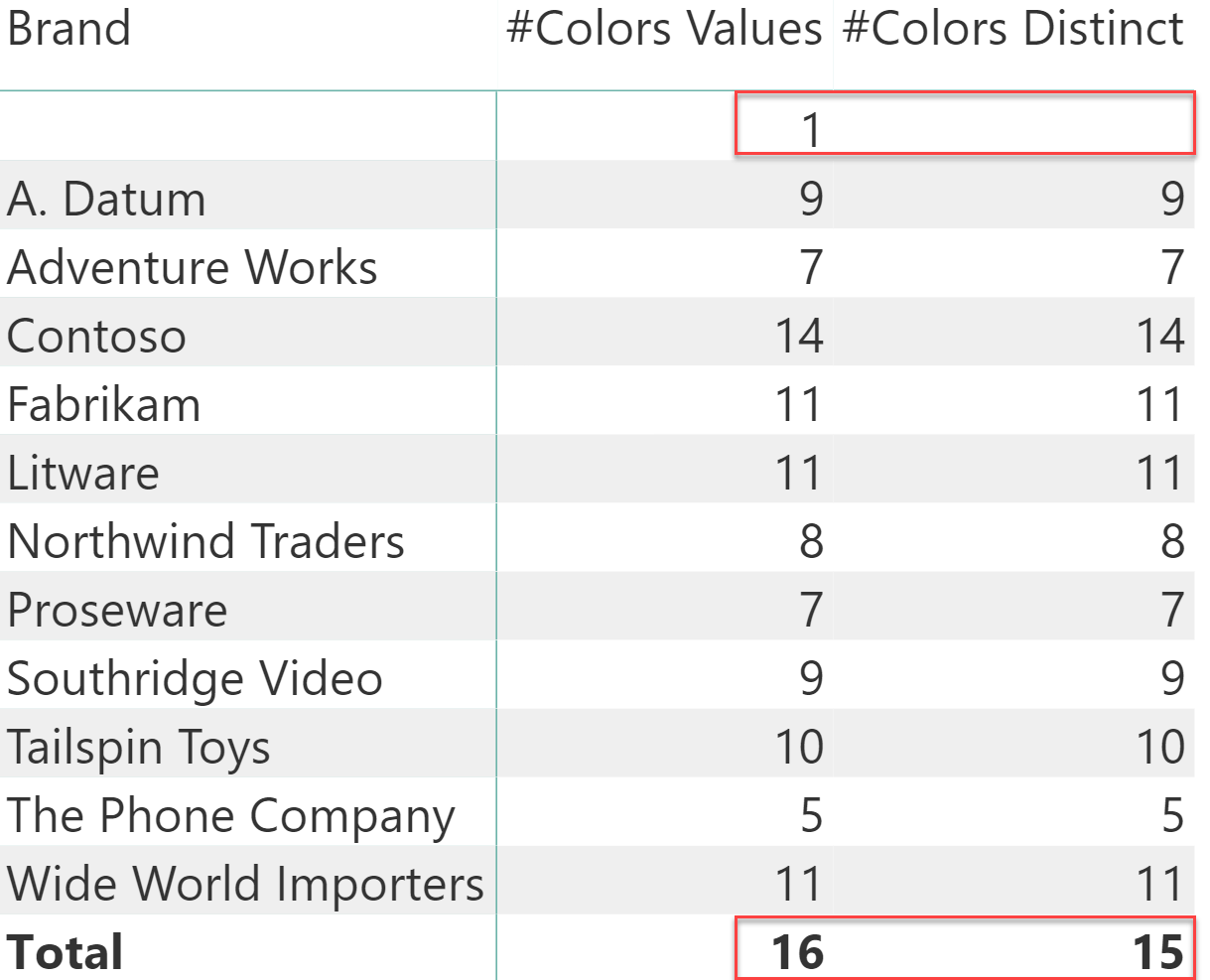As you see, two lines in the report are now different. A new line with a blank brand has appeared at the top, and the grand total shows different values: VALUES returns 16 colors, whereas DISTINCT only returns 15 colors.

This is what happened: by removing products, there are now many orphaned rows in Sales with a value for Sales[ProductKey] that does not match any row in Product. In other words, the relationship is now invalid. If a relationship is invalid, the VertiPaq engine automatically adds a row containing BLANK in every column of the table on the one-side of the relationship – in this case to the Product table. Then, it links all the orphaned rows on the many-side to the blank row on the one-side. Therefore, all the different values of Sales[ProductKey] with no corresponding row in Product are linked to the single blank row.

In the following figure, you can see a visual representation of the blank row.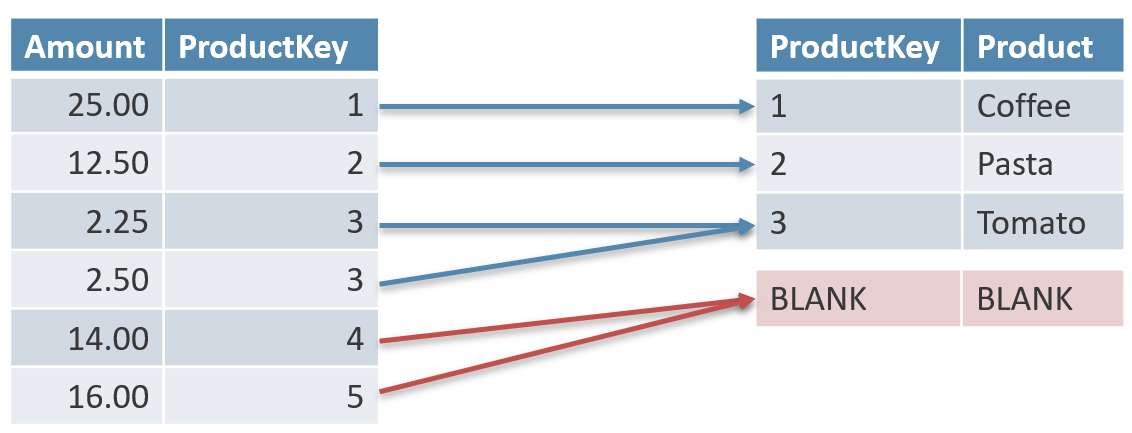The blank row is special. Some functions consider it as a regular row, whereas other functions do not consider it at all. For instance, the VALUES function treats the blank row as a regular row, returning it. On the other hand, DISTINCT never returns the blank row, limiting its result to rows physically loaded in the table.

This is the reason why counting VALUES and DISTINCT produces different results. VALUES reports one additional row (the blank row), whereas DISTINCT does not. If the relationship is valid, that is if all the rows in Sales contain a value that matches one row in Product, then the two functions behave identically.

A good DAX developer knows the functions that consider the blank row and the ones that ignore that same special row. For example, imagine you just want to count the number of products. A quick solution is the following:

```#Products := COUNTROWS ( 'Product' )
```

But used in the previous matrix, this count displays a glitch.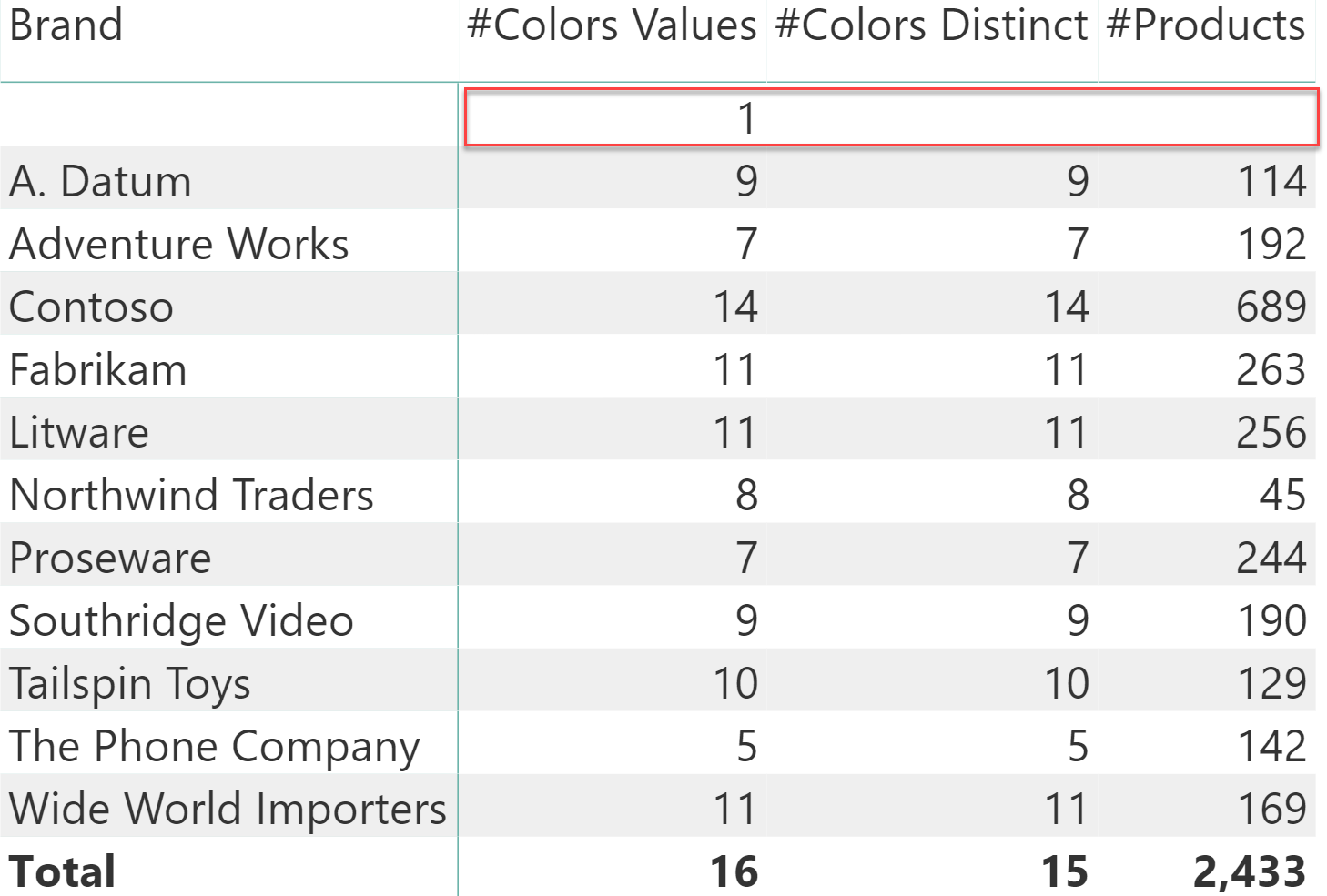You can note that for the blank row, the number of products is missing. The thing is – when you reference a table – the blank row is never returned, as if it were not part of the table. If you want to consider the blank row as a valid row, then you need to perform the counting in a different way:

```#Products Values := COUNTROWS ( VALUES ( 'Product' ) )
```

The next figure highlights the difference between these two measures.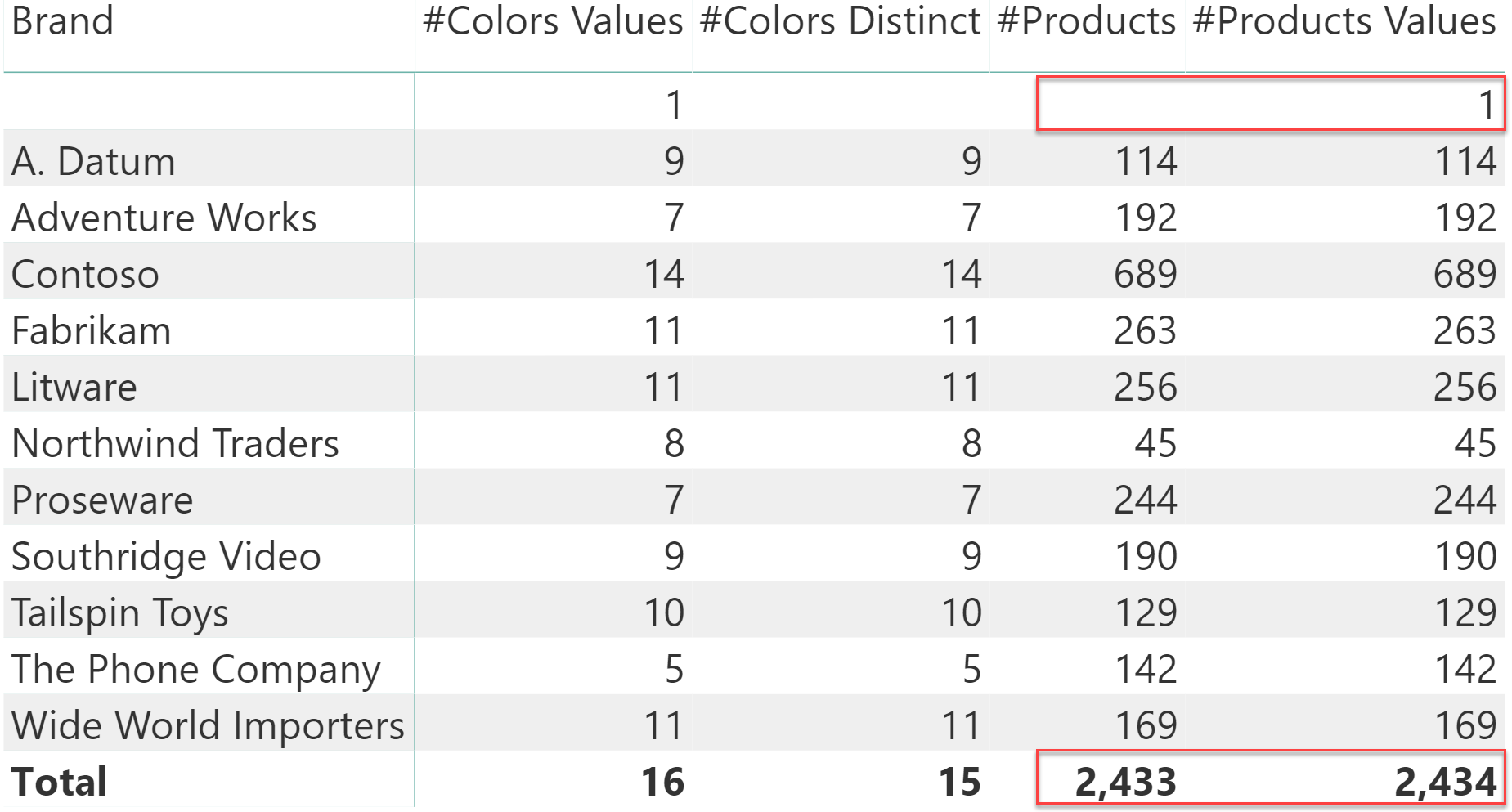There are several scenarios where the difference is important. For example, one might want to compute the number of products of each brand divided by the number of products of all brands. This is possible with a simple calculation like the following:

```Perc #Prods :=
DIVIDE (
COUNTROWS ( 'Product' ),
COUNTROWS ( ALL ( 'Product' ) )
)
```

Despite looking very simple, this code is glitched. If used in a report, it produces a percentage that does not add up to 100%.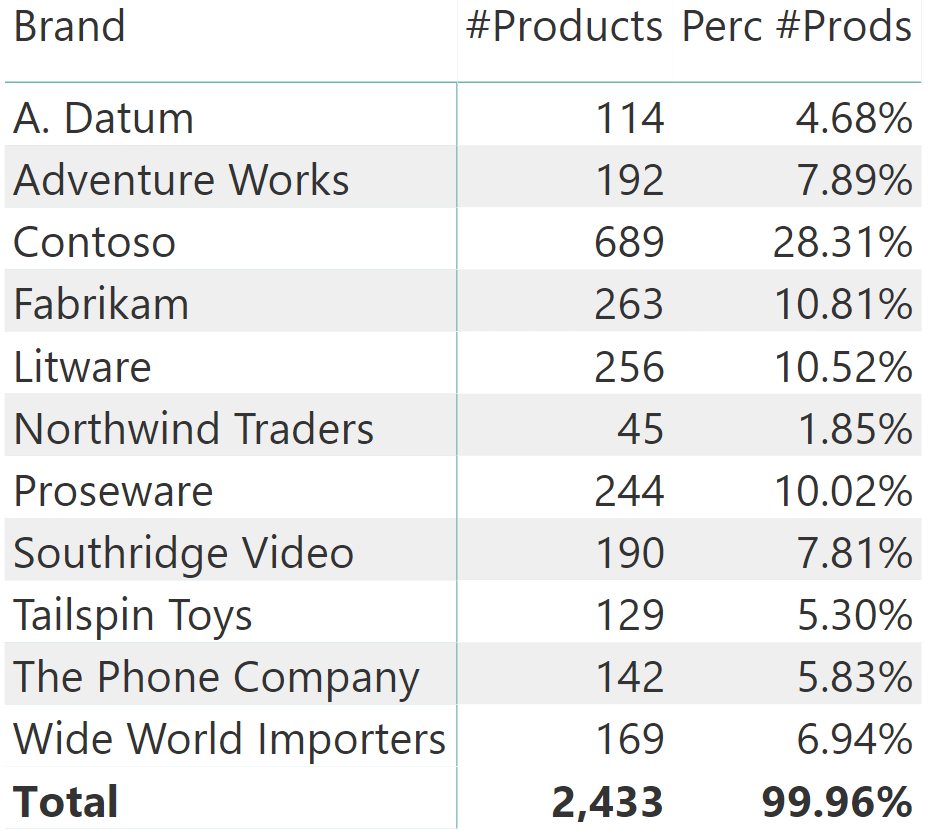The reason is that at the numerator, we used just the table reference Product which does not take into account the blank row. At the denominator on the other hand, we used ALL ( Product ). ALL considers the blank row as valid. Therefore, the numerator is 2,433 and the denominator reports 2,434 leading to an incorrect calculation. The issue can be corrected by changing either the numerator using VALUES, or the denominator using ALLNOBLANKROW. Contrary to ALL, ALLNOBLANKROW does not return the blank row:

```Perc #Prods Values :=
DIVIDE (
COUNTROWS ( VALUES ( 'Product' ) ),
COUNTROWS ( ALL ( 'Product' ) )
)

Perc #Prods Distinct :=
DIVIDE (
COUNTROWS ( DISTINCT ( 'Product' ) ),
COUNTROWS ( ALLNOBLANKROW ( 'Product' ) )
)
```

You can see the results in the following figure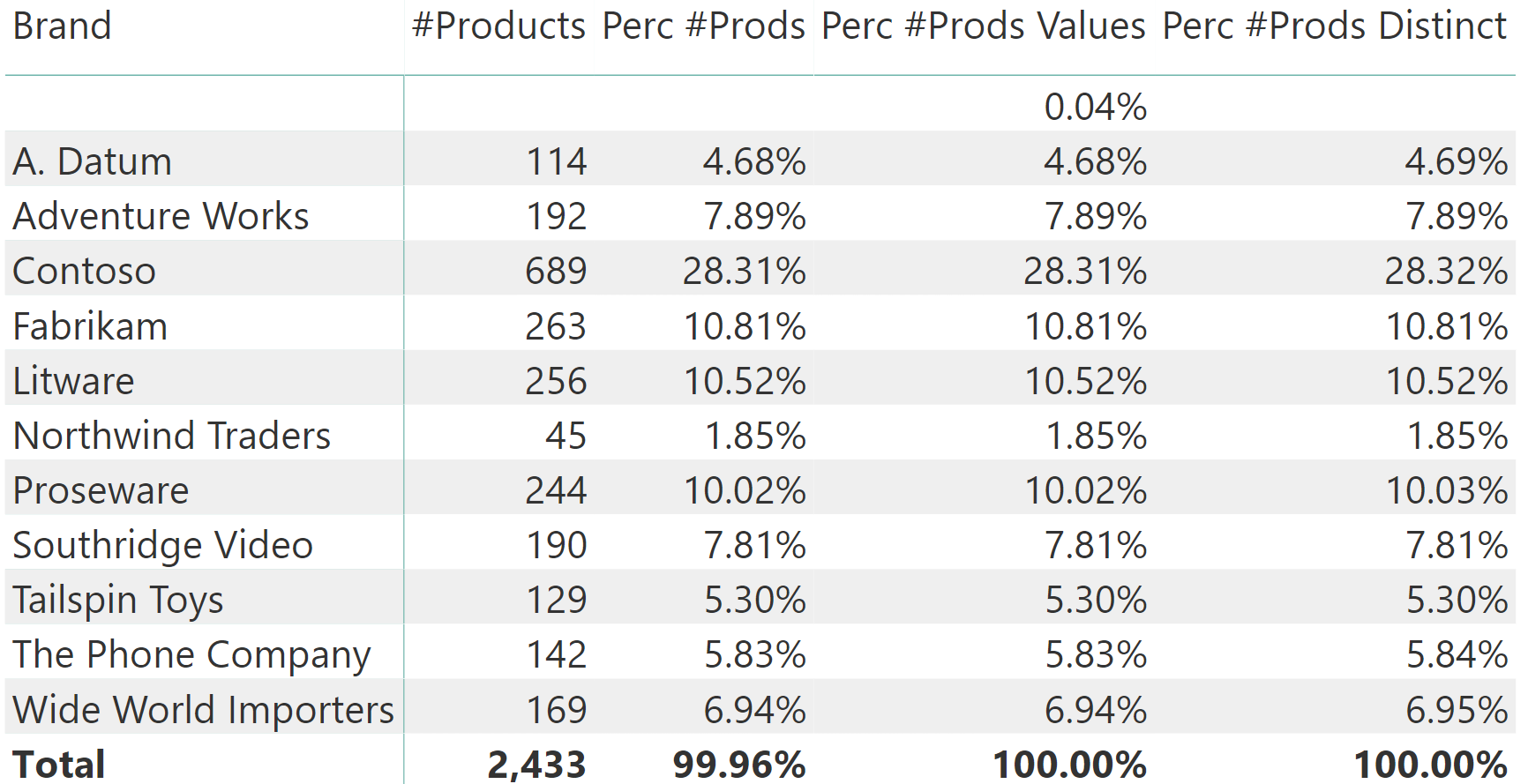Beware that all these calculations provide slightly different results. Choosing one against the other is a very delicate topic that should be carefully evaluated with users, so as to validate the results.

As a rule, one should never work with invalid relationships. Validating relationships is an important part of the data quality assurance process. So in case you face a model that might contain invalid relationships, then it is mandatory that you check all your measures. You want to verify whether your calculations are considering – or not – the blank row as part of their internal processing.

VALUESWhen a column name is given, returns a single-column table of unique values. When a table name is given, returns a table with the same columns and all the rows of the table (including duplicates) with the additional blank row caused by an invalid relationship if present.

`VALUES ( <TableNameOrColumnName> )`

DISTINCTReturns a one column table that contains the distinct (unique) values in a column, for a column argument. Or multiple columns with distinct (unique) combination of values, for a table expression argument.

`DISTINCT ( <ColumnNameOrTableExpr> )`

BLANKReturns a blank.

`BLANK ( )`

ALLCALCULATE modifier

Returns all the rows in a table, or all the values in a column, ignoring any filters that might have been applied.

`ALL ( [<TableNameOrColumnName>] [, <ColumnName> [, <ColumnName> [, … ] ] ] )`

ALLNOBLANKROWCALCULATE modifier

Returns all the rows except blank row in a table, or all the values in a column, ignoring any filters that might have been applied.

`ALLNOBLANKROW ( <TableNameOrColumnName> [, <ColumnName> [, <ColumnName> [, … ] ] ] )`